# Math in Focus Grade 2 Chapter 17 Answer Key Graphs and Line Plots

This handy Math in Focus Grade 2 Workbook Answer Key Chapter 17 Graphs and Line Plots detailed solutions for the textbook questions.

## Math in Focus Grade 2 Chapter 17 Answer Key Graphs and Line Plots

Challenging Practice

Tricia read a story book. She recorded the number of pages she read every day for 3 days. She drew a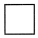for every 2 pages she read.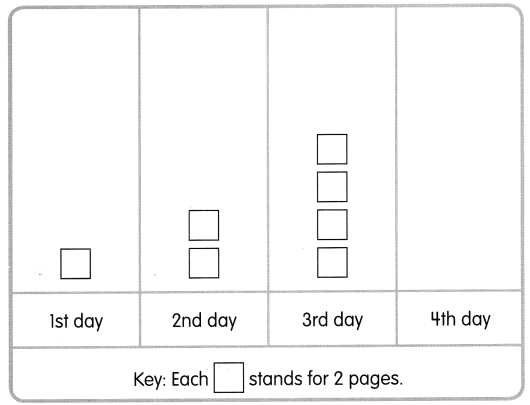The number of pages Tricia read follows a pattern. If this pattern continues, how many pages will Tricia read on the 4th day?
Tricia will read ____________ pages on the 4th day.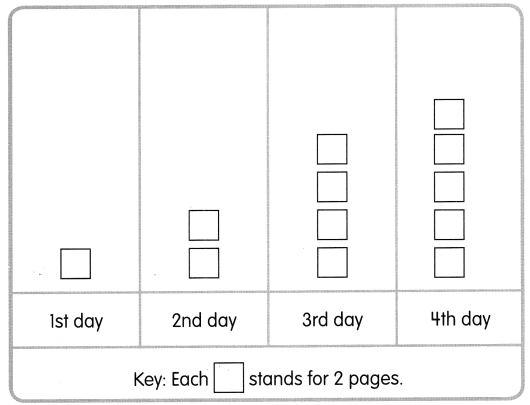Explanation:
She recorded the number of pages she read every day for 3 days
She drew afor every 2 pages she read
The number of pages Tricia read follows a pattern
If this pattern continues Tricia will read 8 + 2 = 10 pages on the 4th day
I drew 5to represent 10 pages as eachstands for 2 pages.

Problem Solving

The graph shows the number of points 5 children scored on a science test. The total score for the test is 40. Complete the graph using the information given.

Ariel scored all the points she could. Tyrone scored 8 points less than Ariel. Nicole and Vera had the same number of points.
Points Scored on a Science Test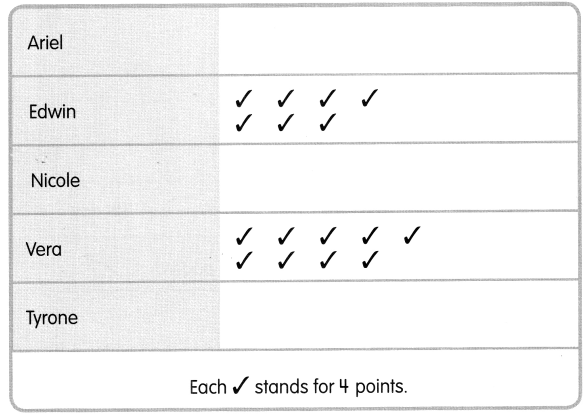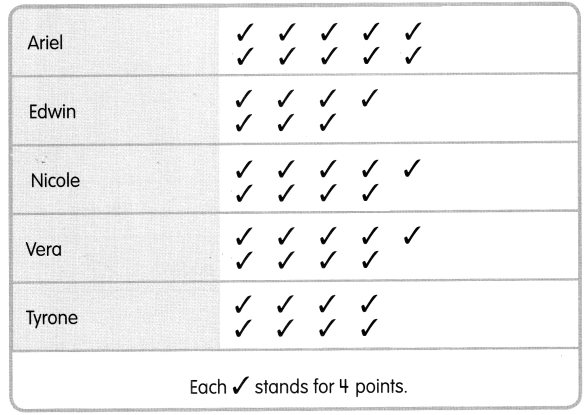Explanation:
I completed the picture graphs using the given information
The graph shows the number of points 5 children scored on a science test. The total score for the test is 40
Ariel scored all the points she could
Ariel scored 40 points
Each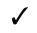stands for 4 points
40 ÷ 4 = 10
So, i drew 10for Ariel
Tyrone scored 8 points less than Ariel
That is 2less than Ariel
So, Tyrone has 8Nicole and Vera had the same number of points
Vera have 9that is 36 points
So, Nicole will also have 9that is 36 points.

Chapter Review/Test

Vocabulary

Fill in the blanks with words from the box.

key
picture graph
tally chart

Question 1.
The ___________ shows what a picture or symbol stands for.

The key shows what a picture or symbol stands for.

Question 2.
A ___________ shows data using pictures or symbols.
A picture graph shows data using pictures or symbols.

Question 3.
You record the number of things in a _____________.
You record the number of things in a tally chart.

Concepts and Skills

Question 4.
Tammy went to a bird park. Help her make a tally chart of the birds she saw on page 202.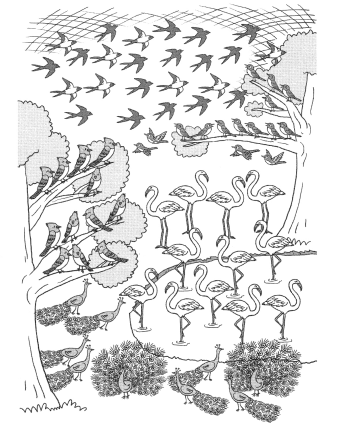Tally Chart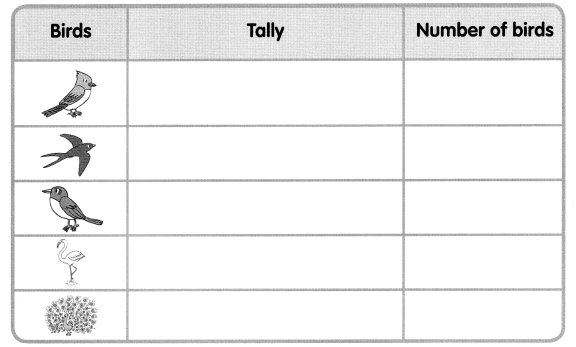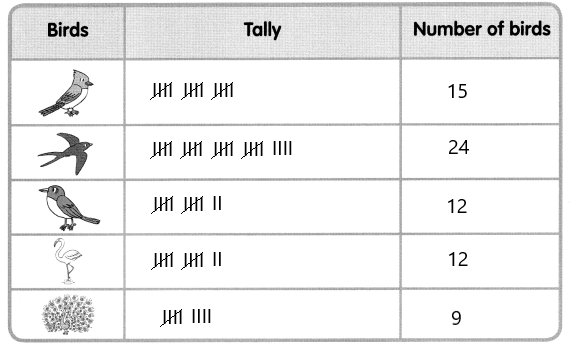Explanation:
Tammy went to a bird park
I counted the number of birds of each type and drew tally marks for the numbers.

Question 5.
Then complete the picture graph of the data in exercise 4. Give it a title.
Title: ______________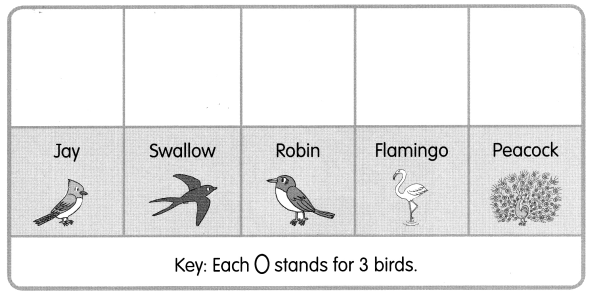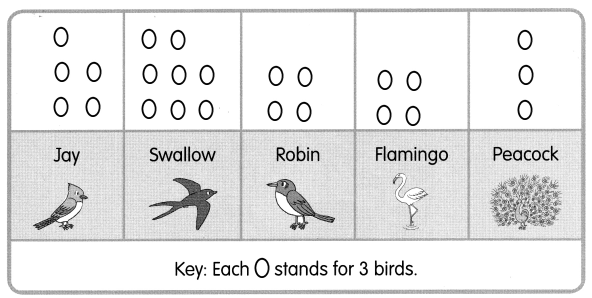Explanation:
I drew a picture graph using the key
I used the key, each picture key stands for 3 birds
So, i drew the pictures to represent the number of birds of each type
There are 15 Jays, 24 Swallows, 12 Robins, 12 Flamingos and 9 peacocks.

The graph shows how much money each child has saved in a month.
Money Saved in One Month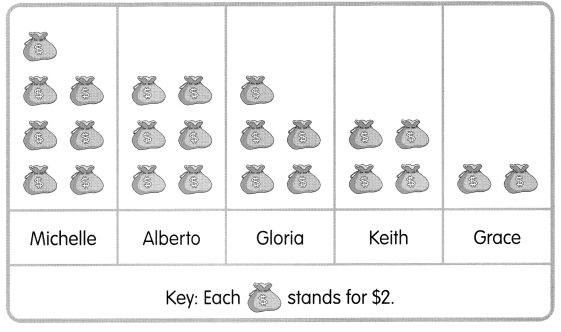Use the graph to find the missing numbers.

Question 6.
How much has Keith saved?
There are _________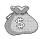for Keith.
________ × $_________ =$___________
Keith has saved $___________ Answer:$8

Explanation:
There are 4for Keith.
4 × $2 =$ 8
Keith has saved $8. Question 7. How much have Michelle and Alberto saved in all? There are 7for Michelle. __________ ×$___________ = $_________ There are 6for Alberto. _________ ×$_________ = $__________$________ + $_________ =$___________
Michelle and Alberto saved $_________ in all. Answer:$ 26

Explanation:
There are 7for Michelle

7 × $2 =$ 14
There are 6for Alberto.
6 × $2 =$ 12
$14 +$ 12 = $26 Michelle and Alberto saved$ 26 in all

Question 8.
How much more has Michelle saved than Gloria?
There are 7for Michelle.
__________ × $___________ =$_________
There are 5for Gloria.
_________ × $_________ =$__________
$________ –$_________ = $___________ Michelle has saved$_________ more than Gloria.
$4 Explanation: There are 7for Michelle. 7 ×$ 2 = $14 There are 5for Gloria. 5 ×$ 2 = $10$ 14 – $10 =$ 4
Michelle has saved $4 more than Gloria. Question 9. Grace has 2. How much less has she saved than Gloria? _________ ×$_________ = $__________$________ – $_________ =$___________
Grace has saved $___________ less than Gloria. Answer:$ 6

Explanation:
Grace has 22 x $2 =$ 4
Gloria has 55 × $2 =$ 10
$10 –$ 4 = $6 Grace has saved$ 6 less than Gloria.

The chart shows the number of beads that each child used to make a bracelet.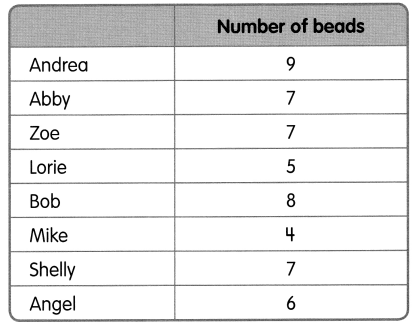Use the data to complete the bar graph below. Give your graph a title.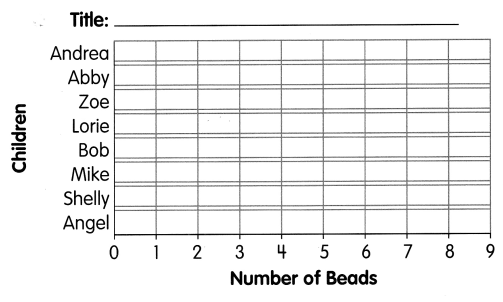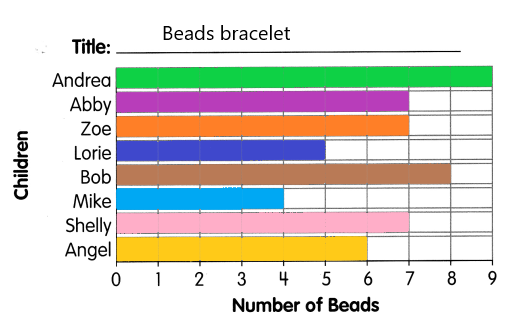Make a line plot using the data on the bar graph on page 205. Then answer the questions.

Question 10.
How many children used 6 beads?
1 child

Explanation:

Question 11.
How many children used fewer than 7 beads?
3 children

Explanation:

Question 12.
8

Explanation:

Question 13.
How many children used more than 7 beads?
2 children

Explanation: